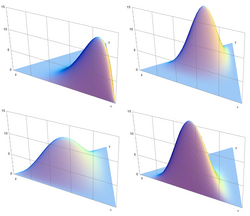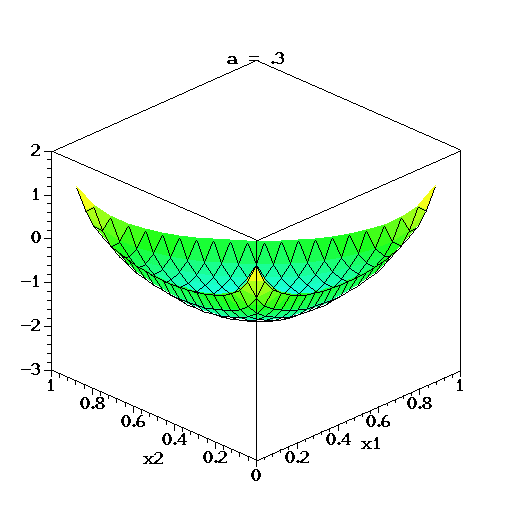Sunday, October 28, 2007

Visualizing the Dirichlet

Last week, while working with Foster Provost and Xiahoan Zhang, one of our PhD students, we were trying to understand the internals of the Latent Dirichlet Allocation.

In particular, we were getting strange results from the LDA-C program by David Blei, and we wanted to figure out what we were doing wrong. The first suspects were the parameter values. We wanted to see how the values of the different parameters affect the behavior of the technique.

The conceptual model of LDA is pretty simple. We have a Dirichlet distribution, parameterized by a k-dimensional vector alpha (k is the number of topics). The Dirichlet distribution allows us to draw k-dimensional random vectors theta_i, one for each document in the collection. The vector theta_i represents the topic mixture within the document. For example, for 3 topics (say, art, technology, and sports), a vector theta_i = (0.2, 0.5, 0.3), means that the document i is 20% about art, 50% about technology, and 30% about sports. Then, each topic is modeled as a random distribution of words, representing the relative frequency of each word within the given topic.

The "alpha" parameter of the Dirichlet is crucial, as it defines the distribution of the theta_i vectors or, in other words, the "distribution of topic distributions".

After looking at some visualizations of the Dirichlet, the demonstrated shapes looked strange. Most of the visualizations of the Dirichlet (e.g., on Wikipedia) showed that the mass of the density distribution is around the "center" of the simplex. For example for a Dirichlet with three topics (image from Wikipedia):most of the density was around the area (0.33, 0.33, 0.33). At the same time, very little mass was allocated at the "corners" of the simplex. Such a shape implied that the majority of the theta vectors drawn from such a distribution would be vectors that have mix many topics within a document. At the same time, very few documents would be generated with only one topic.

This was a counterintuitive modeling choice. Why do we want to force most documents to have many topics and not have the majority of documents to have only a small number of topics? Something seemed wrong.

To understand better how the Dirichlet density function is allocated in the simplex, I pulled my favorite Maple, and started coding my visualization code. First, I defined the 3-d Dirichlet density function.
B := (a1, a2, a3) -> (GAMMA(1.0*a1) * GAMMA(1.0*a2) * GAMMA(1.0*a3)) / GAMMA(1.0*a1+1.0*a2+1.0*a3);

Dir := (x1, x2, a1, a2, a3) -> (x1^(a1-1)) * (x2^(a2-1)) * ( (1-x1-x2)^(a3-1)) /B(a1,a2,a3) ;

Then, I plotted the log of the density in an animated 3d plot. Since I just wanted to see how the value of the vector alpha change the distribution, I decided to keep all the vector elements to alpha equal to each other, and vary them to have values from 0.3 to 2.0.

with(plots);

plotsetup(gif, plotoutput=LogDirichletDensity-alpha_0.3_to_alpha_2.0.gif);

animate ( plot3d, [eval(log(Dir(x1, x2, a1, a2, a3)), {a1=a, a2=a, a3=a}), x1=0.00..1, x2=0.00..1, axes=BOXED, grid=[25,25], gridstyle=triangular, orientation=[-135, 60], shading=zhue, contours=20, style=surfacecontour, view=-3..2 ], a=0.3..2.0, frames=100);

The result was pretty revealing:When the values of alpha are below 1.0, the majority of the probability mass is in the "corners" of the simplex, generating mostly documents that have a small number of topics. When the values of alpha are above 1.0, the majority of the generated documents tend to contain all the topics. (Yes, I am pretty sure that any people with experience in Bayesian statistical modeling who are reading this post are yawning by now. But this is my blog and I will write things that I find interesting.)

So, for general topic collections, and traditional "topical clustering" tasks, it seems best to drive the LDA inference towards configurations that have low alpha values, so that each document contains a small number of topics.

Someone might ask, when we would want at all to examine configurations where alpha is higher than 1.0? Can such configurations be useful at all? Well, there are cases when documents tend to contain frequently a mixture of topics. In product reviews, the reviewers tend to cover a large number of product features (e.g., for a digital camera they will talk about the lenses, about the image quality, about battery life, and so on). Hotel reviews on TripAdvisor also exhibit a similar structure. Similarly, in feedback postings in the reputation systems of eBay and of Amazon, the buyers tend to give feedback about various characteristics of the merchant with whom they transacted (how fast was the delivery, whether the product was accurately described, and so on). In these cases, LDA tends to work best when the values of alpha are above 1.0. Otherwise the resulting topics do not make much sense.